## How to Calculate and Solve for the Height, Base Edge and Volume of a Square Pyramid | The Calculator Encyclopedia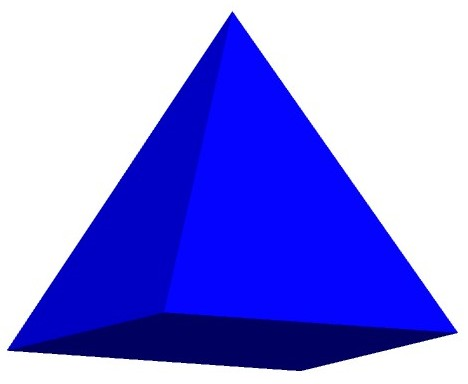The image above represents a square pyramid.
To compute the volume of a square pyramid requires two essential parameters which are the base edge and height of the square pyramid.

The formula for computing the volume of a square pyramid is:

V = ha² / 3

Where:
V = Volume of the Square Pyramid
a = Base edge of the Square Pyramid
h = Height of the Square Pyramid

Let’s solve an example
Find the volume of a square pyramid with a base edge of 6 cm and a height of 11 cm.

This implies that:
a = base edge of the square pyramid = 6
h= = height of the square pyramid = 11

V = ha² / 3
V = 11(6)² / 3
V = 11(36) / 3
V = 396 / 3
V = 132

Therefore, the volume of the square pyramid is 132 cm3.

Calculating the Base edge of a square pyramid when Volume and Height are Given

The formula is a = √(3V / h)

Where;
V = Volume of the Square Pyramid
a = Base edge of the Square Pyramid
h = Height of the Square Pyramid

Let’s solve an example:
Find the base edge of a square pyramid with a volume of 50 cm3 and a height of 20 cm.

This implies that;
V = Volume of the square pyramid = 50 cm3
h  = height of the square pyramid = 20 cm

a = √(3V / h)
a = √(3(50) / 20)
a = √(150 / 20)
a = √7.5
a = 2.739

Therefore, the base edge of the square pyramid is 2.739 cm.

Calculating the height of a square pyramid when Volume and Base edge are Given

The formula is h = 3V / a2

Where;
a = Base edge of the square pyramid
V = Volume of the Square Pyramid
h = Height of the Square Pyramid

Let’s solve an example:
Find the height of a square pyramid with a volume of  250 cm3  and a base edge of 7 cm2

This implies that;
V = Volume of the square pyramid = 250 cm3
a = Base edge of the square pyramid = 7 cm

h =  3V /
h = 3(250) /
h = 750 / 49
h = 15.306

Therefore, the height of the square pyramid is 15.306 cm.

## How to Calculate the Volume, Base Area and Height of a Prism | The Calculator Encyclopedia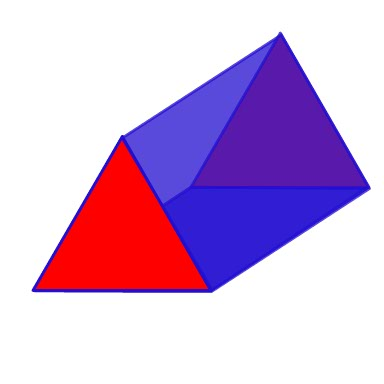The image above represents a prism.
To compute the volume of a prism requires two essential parameters which are the base area and height of the prism.

The formula for computing the volume of a prism is:

V = Ah

Where:
V = Volume of the Prism
A = Base area of the Prism
h = Height of the prism

Let’s solve an example
Find the volume of a prism with a base area of 30 cm2 and a height of 8 cm.

This implies that:
A = base area of the prism = 30
h= = height of the prism = 8

V = Ah
V = 30 x 8
V = 240

Therefore, the volume of the prism is 240 cm3.

Calculating the Base area of a prism when Volume and Height are Given

The formula is A = V / h

Where;
V = Volume of the Prism
A = Base area of the Prism
h = Height of the Prism

Let’s solve an example:
Find the base area of a prism with a volume of 300 cm3 and a height of 12 cm.

This implies that;
V = Volume of the prism = 300 cm3
h  = height of the prism = 12 cm

A =  V / h
A = 300 / 12
A = 25

Therefore, the base area of the prism is 25 cm2.

Calculating the height of a prism when Volume and Base area are Given

The formula is h = V / A

Where;
A = Base area of the Prism
V = Volume of the Prism
h = Height of the Prism

Let’s solve an example:
Find the height of a prism with a volume of  280 cm3  and a base area of 35 cm2

This implies that;
V = Volume of the prism = 280 cm3
A = Base area of the prism = 35 cm2

h =  V / A
h = 280 / 35
h = 8

Therefore, the height of the prism is 8 cm.

## How to Calculate the Volume, Length, Width and Height of a Cuboid | Nickzom Calculator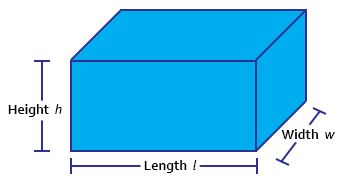The image above represents a cuboid.
To compute the volume of a cuboid requires three essential parameters which are the length, width and height of the cuboid.

The formula for computing the volume of a cuboid is:

V = lwh

Where:
V = Volume of the Cuboid
l = Length of the Cuboid
w = Width of the Cuboid
h = Height of the Cuboid

Let’s solve an example
Find the volume of a cuboid with a length of 6 cm, width of 2 cm and a height of 10 cm.

This implies that:
l = length of the cuboid = 6
w = width of the cuboid = 2
h= = height of the cuboid = 10

V = lwh
V = 6 x 2 x 10
V = 120

Therefore, the volume of the cuboid is 120 cm3.

Calculating the Length of a cuboid when Volume, Width and Height are Given

The formula is l = V / (w)(h)

Where;
V = Volume of the Cuboid
l = Length of the Cuboid
w = Width of the Cuboid
h = Height of the Cuboid

Let’s solve an example:
Find the length of a cuboid with a volume of 440 cm3 , a width of 5 cm and a height of 11 cm.

This implies that;
V = Volume of the cuboid = 440 cm3
w = width of the cuboid = 5 cm
h  = height of the cuboid = 11 cm

l =  V / (w)(h)
l = 440 / (5)(11)
l = 440 / 55
l = 8 cm

Therefore, the length of the cuboid is 8 cm.

Calculating the Width of a cuboid when Volume, Length and Height are Given

The formula is w = V / (l)(h)

Where;
V = Volume of the Cuboid
l = Length of the Cuboid
w = Width of the Cuboid
h = Height of the Cuboid

Let’s solve an example:
Find the width of a cuboid with a volume of  180 cm3 , a length of 6 cm and a height of 10 cm

This implies that;
V = Volume of the cuboid = 180 cm3
l = length of the cuboid = 6 cm
h  = height of the cuboid = 10 cm

w =  V / (l)(h)
w = 180 / (6)(10)
w = 180 / 60
w = 3 cm

Therefore, the width of the cuboid is 3 cm.

Calculating the Height of a cuboid when Volume, Length and Width are Given

The formula is h = V / (l)(w)

Where;
V = Volume of the Cuboid
l = Length of the Cuboid
w = Width of the Cuboid
h = Height of the Cuboid

Let’s solve an example:
Find the height of a cuboid with a volume of 195 cm3 , a length of 5 cm and a width of 3 cm

This implies that;
V = Volume of the cuboid = 195 cm3
l = length of the cuboid = 5 cm
w  = width of the cuboid = 3 cm

h =  V / (l)(w)
h = 195 / (5)(3)
h = 195 / 15
h = 13 cm

Therefore, the height of the cuboid is 13 cm.

## How to Calculate and Solve for the Area, Length, Width and Height of a Cuboid | Nickzom Calculator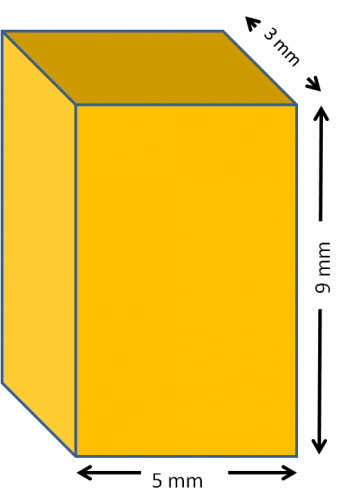The image above represents a cuboid.
To compute the area of a cuboid requires three essential parameters which are the length, width and height of the cuboid.

The formula for computing the area of a cuboid is:

A = 2(lw + lh + wh)

Where:
A = Area of the Cuboid
l = Length of the Cuboid
w = Width of the Cuboid
h = Height of the Cuboid

Let’s solve an example
Find the area of a cuboid with a length of 5 cm, width of 3 cm and a height of 9 cm.

This implies that:
l = length of the cuboid = 5
w = width of the cuboid = 3
h= = height of the cuboid = 9

A = 2(lw + lh + wh)
A = 2(5 x 3 + 5 x 9 + 3 x 9)
A = 2(87)
A = 174

Therefore, the area of the cuboid is 174 cm2.

Calculating the Length of a cuboid when Area, Width and Height are Given

The formula is l = A – 2(w)(h) / 2(w) + 2(h)

Where;
A = Area of the Cuboid
l = Length of the Cuboid
w = Width of the Cuboid
h = Height of the Cuboid

Let’s solve an example:
Find the length of a cuboid with an area of 140 cm2 , a width of 4 cm and a height of 12 cm

This implies that;
A = Area of the cuboid = 140 cm2
w = width of the cuboid = 4 cm
h  = height of the cuboid = 12 cm

l =  A – 2(w)(h) / 2(w) + 2(h)
l = 140 – 2(4)(12) / 2(4) + 2(12)
l = 140 – 96 / 8 + 24
l = 44 / 32
l = 1.375 cm

Therefore, the length of the cuboid is 1.375 cm.

Calculating the Width of a cuboid when Area, Length and Height are Given

The formula is w = A – 2(l)(h) / 2(l) + 2(h)

Where;
A = Area of the Cuboid
l = Length of the Cuboid
w = Width of the Cuboid
h = Height of the Cuboid

Let’s solve an example:
Find the width of a cuboid with an area of 200 cm2 , a length of 5 cm and a height of 12 cm

This implies that;
A = Area of the cuboid = 200 cm2
l = length of the cuboid = 5 cm
h  = height of the cuboid = 12 cm

w =  A – 2(l)(h) / 2(l) + 2(h)
w = 200 – 2(5)(12) / 2(5) + 2(12)
w = 200 – 120 / 10 + 24
w = 80 / 34
w = 2.353 cm

Therefore, the width of the cuboid is 2.353 cm.

Calculating the Height of a cuboid when Area, Length and Width are Given

The formula is h = A – 2(l)(w) / 2(l) + 2(w)

Where;
A = Area of the Cuboid
l = Length of the Cuboid
w = Width of the Cuboid
h = Height of the Cuboid

Let’s solve an example:
Find the height of a cuboid with an area of 300 cm2 , a length of 6 cm and a width of 2 cm

This implies that;
A = Area of the cuboid = 300 cm2
l = length of the cuboid = 6 cm
w  = width of the cuboid = 2 cm

h =  A – 2(l)(w) / 2(l) + 2(w)
h = 300 – 2(6)(2) / 2(6) + 2(2)
h = 300 – 24 / 12 + 4
h = 276 / 16
h = 17.25 cm

Therefore, the height of the cuboid is 17.25 cm.

## How to Calculate and Solve for the Volume, Radius and Height of a Cylinder | Nickzom Calculator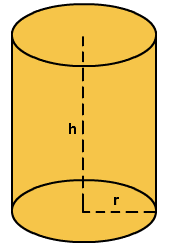The image above represents a cylinder.
To compute the volume of a cylinder requires two essential parameters which are the radius and height of the cylinder.

The formula for computing the volume of a cylinder is:

V = πr2h

Where:
V = Volume of a cylinder
r = radius of the cylinder
h = height of the cylinder

Let’s solve an example
Find the volume of a cylinder with a radius of 3 cm and a height of 5 cm.

This implies that:
r = radius of the cylinder = 3
h = height of the cylinder = 5

V = πr2h
V = 3.142 x 32 x 5
V = 141.39

Therefore, the volume of the cylinder is 141.39 cm3.

Calculating the Height of a cylinder when Volume and Radius is Given

The formula is h = V / πr2

Where;
V = Volume of a cylinder
r =  radius of the cylinder
h = height of the cylinder

Let’s solve an example:
Find the height of a cylinder with a volume of 300 cm3 and a radius of 3 cm

This implies that;
V = Volume of the cylinder = 300 cm3
r  = radius of the cylinder = 3 cm

h = V / πr2
h = 300 / 3.142(3)2
h = 300 / 28.278
h = 10.61
Therefore, the height of the cylinder is 10.61 cm.

Calculating the Radius of a cylinder when Volume and Height is Given

The formula is r = √(V / πh)

Where;
V = Volume of a cylinder
r =  radius of the cylinder
h = height of the cylinder

Let’s solve an example:
Find the radius of a cylinder with a volume of 200 cm3 and a height of 5 cm

This implies that;
V = Volume of the cylinder = 200 cm3
h  = height of the cylinder = 5 cm

r = √(V / πh)
r = √(200 / 3.142(5))
r = √(200 / 15.71)
r = √12.73
r = 3.57

Therefore, the radius of the cylinder is 3.57 cm.

## How to Calculate the Area, Radius and Height of a Cylinder | Nickzom Calculator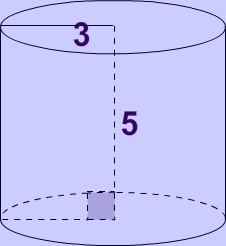The image above represents a cylinder.
To compute the area of a cylinder requires two essential parameters which are the radius and height of the cylinder.

The formula for computing the area of a cylinder is:

A = 2πrh + 2πr2

Where:
A = Area of a cylinder
r = radius of the cylinder
h = height of the cylinder

Let’s solve an example
Find the area of a cylinder with a radius of 3 cm and a height of 5 cm.

This implies that:
r = radius of the cylinder = 3
h = height of the cylinder = 5

A = 2πrh + 2πr2
A = 2(3.142) x 3 x 5 + 2(3.142) x 32
A = 150.82

Therefore, the area of the cylinder is 150.82 cm2.

Calculating the Height of a cylinder when Area and Radius is Given

The formula is h =  A/ 2πr – r

Where;
A = Area of a cylinder
r =  radius of the cylinder
h = height of the cylinder

Let’s solve an example:
Find the height of a cylinder with an area of 600 cm² and a radius of 5 cm

This implies that;
A = Area of the cylinder = 600 cm²
r  = radius of the cylinder = 5 cm

h = A / 2πr – r
h = 600 / 2(3.142)(5) – 5
h = 600 / 31.42 – 5
h = 19.10 – 5
h = 14.10

Therefore, the height of the cylinder is 14.10 cm.

## How to Calculate and Solve for the Area and Length of a Parallelogram | The Calculator EncyclopediaThe image above represents a parallelogram.
To compute the area of a parallelogram requires two essential parameters which are the base and height of the parallelogram.

The formula for computing the area of a parallelogram is:

A = b x h

Where:
A = Area of a parallelogram
b = base of the parallelogram
h = height of the parallelogram

Let’s solve an example
Find the area of a parallelogram with a base of 12 cm and a height of 4 cm.

This implies that:
b = base of the parallelogram = 12
h = height of the parallelogram = 4

A = b x h
A = 12 x 4
A = 48

Therefore, the area of the parallelogram is 48 cm2.

Calculating the base of a parallelogram when Area and Height is Given

The formula is b = A / h

Where;
A = Area of a parallelogram
b = base of the parallelogram
h = height of the parallelogram

Let’s solve an example:
Find the base of a parallelogram with an area of 40 cm² and a height of 8 cm

This implies that;
A = Area of the parallelogram = 40 cm²
h = height of the parallelogram = 8 cm

b = A / h
b = 40 / 8
b = 5

Therefore, the base of the parallelogram is 5 cm.

Calculating the height of a parallelogram when Area and Base is Given

The formula is h = A / b

Where;
A = Area of a parallelogram
b = base of the parallelogram
h = height of the parallelogram

Let’s solve an example:
Find the height of a parallelogram with an area of 60 cm² and a base of 6 cm

This implies that;
A = Area of the parallelogram = 60 cm²
b = base of the parallelogram = 6 cm

h = A / b
h = 60 / 6
h = 10

Therefore, the height of the parallelogram is 10 cm.

## How to Calculate and Solve for the Perimeter and Length of a Square | The Calculator Encyclopedia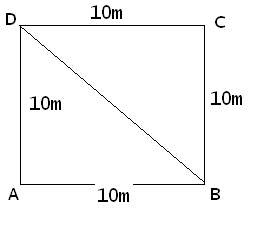The image above represents a square.
To compute the perimeter of a square requires one essential parameter which is the length of one side of a square.

The formula for computing the perimeter of a square is:

P = 4 x l

Where:
P = Perimeter of a Square
l = Length of one side of a Square

Let’s solve an example
Find the perimeter of a square which has a length of 10 m.

This implies that:
l = length of one side of a square = 10

P = 4 x l
P = 4 x 10
P = 40

Therefore, the perimeter of the square is 40 m.

Calculating the Length of a Square when Perimeter is Given

The formula is l = p / 4

Where;
P = Perimeter of a square
l = Length of a square

Let’s solve an example:
Find the length of a square where the perimeter of a square is 100 m.

This implies that;
P = Perimeter of a square = 100 m

l = p / 4
l = 100 / 4
l = 25

Therefore, the length of the square is 25 m.

## How to Calculate and Solve for the Area and Length of a Square | The Calculator Encyclopedia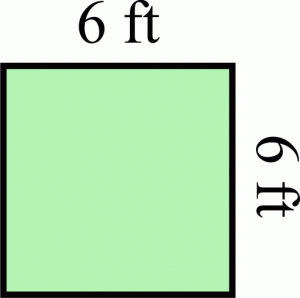The image above represents a square.
To compute the area of a square requires one essential parameter which is the length of one side of a square.

The formula for computing the area of a square is:

A = l2

Where:
A = Area of a Square
l = Length of one side of a Square

Let’s solve an example
Find the area of a square which has a length of 6 ft.

This implies that:
l = length of one side of a square = 6

A = l2
A = 62
A = 6 x 6
A = 36

Therefore, the area of the square is 36 ft2.

Calculating the Length of a Square when Area is Given

The formula is l = √[A]

Where;
A = Area of a square
l = Length of a square

Let’s solve an example:
Find the length of a square where the area of a square is 81 ft2.

This implies that;
A = Area of a square = 81 ft2

l = √[A]
l = √
l = 9

Therefore, the length of the square is 9 ft.